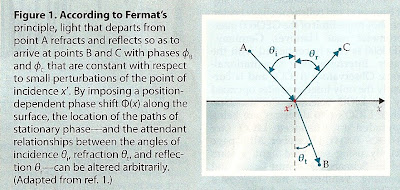## Thursday, November 24, 2011

### A Re-Thinking of Snell's Law?Readers may recall an earlier blog:

http://brane-space.blogspot.com/2011/06/basic-physics-light-and-optics-pt-17.html

in which we examined light and optics in term's of "Snell's Law", which basically says that the ratio of the refractive index in a more dense medium to the refractive index in a less dense medium is the ratio of the velocity of light in the more dense medium to the value in the less dense medium.

Taking into account the two angles: Θ1 = angle of incidence, and Θ2 = angle of refraction, we may write:

n1 sin Θ1 = n2 sin Θ2

This may also be written:

n1 sin Θ1 - n2 sin Θ2 = 0

at the heart of this law is another physical principle, that of Fermat, which says that light will always take the "fastest path", i.e. that light rays travel the fastest path between two points. Interestingly, Richard Feynman in his Caltech 'Lectures on Physics' also showed how Fermat's Principle could apply to mechanics in terms of "the Principle of Least Time". But that's another blog!

Evidently, now - as reported in the most recent issue of Physics Today (Nov., p. 12) Harvard researchers are posing questions neither Fermat or Feynman likely considered, such as: How would a light ray's trajectory change if - at the surface of reflection and refraction - it experienced a position-dependent phase shift?

The researchers have combined theory and experiment and, using a specially constructed phase-shifting surface, shown that light can be bent in ways that defy the traditional laws of reflection, and refraction, including Snell's law.

Their underlying premise is that Fermat's principle works not because light is in some kind of a "race" to go from one place to the other, but because (mathematically) the fastest path lies at an extremum, e.g where the derivative dL/dx, of the optical path length with respect to small deviations in the trajectory, is zero.

In the Harvard experiments, it was found that away from the extremum, neighboring trajectories for light cancel each other out. In this case, Fermat's Principle can be recast as a 'principle of stationary phase' instead. In other words, a ray sych as depcited in Fig. 1 which reflects and refracts such that light originating at point A arrives at B and C with phases φ(B) and φ(C) that are constant relative to small perturbations of the point of incidence, x'.

Thus, the light will travel the path for which:

dφ(B)/ dx' = 0

and

dφ(C)/ dx' = 0

Adopting the more generalized interpretation of Fermat's Principle, the Harvard researchers whowed that a position dependent phase shift, say φ(x), imposed at the reflecting-refracting surface can alter the location of stationary phase and, concurrently, the usual optical laws (cited in the previous blog link) for refraction and reflection.

The effect might then be accomodated with suitable modifications to Snell's law such that we now write (as opposed to the earlier simplified version):

n1 sin Θ1 - n2 sin Θ2 = (L/ 2π) dφ/ dx

Similarly, for the law of relection, we'd use:

sin Θ_r - sin Θ_i = (L/ 2π) dφ/ dx

where L denotes the wavelength of light for the particular experiment.

The more nuanced Harvard re-do of the optical laws is well justified since they (based on experiment) introduce a structured phase delay with a gradient along the surface.

Here's something else to ponder: David Deutsch in his The Fabric of Reality, has postulated phase and interference effects (pp. 45-51) that can theoretically reveal the presence of "shadow particles" from parallel universes. If so, perhaps further refinements of the Harvard and other experiments (some as proposed by Deutsch) might reveal if indeed we're able to detect the incursion of such parallel cosmi into our own!